### Still have math questions?

Algebra
Question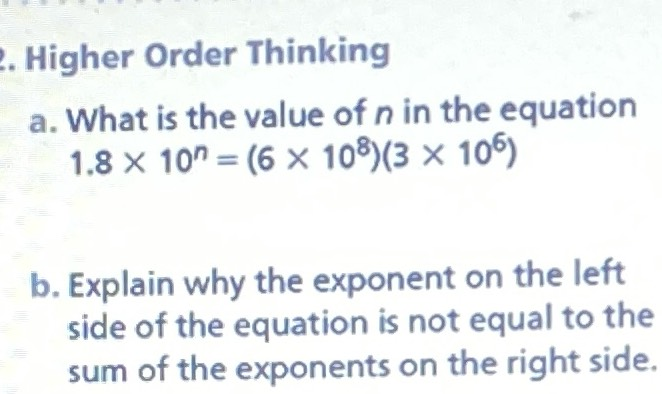Higher Order Thinking

a. What is the value of $$n$$ in the equation

$$1.8 \times 10 ^ { n } = ( 6 \times 10 ^ { 8 } ) ( 3 \times 10 ^ { 6 } )$$

b. Explain why the exponent on the left side of the equation is not equal to the sum of the exponents on the right side.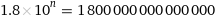ResultSolutions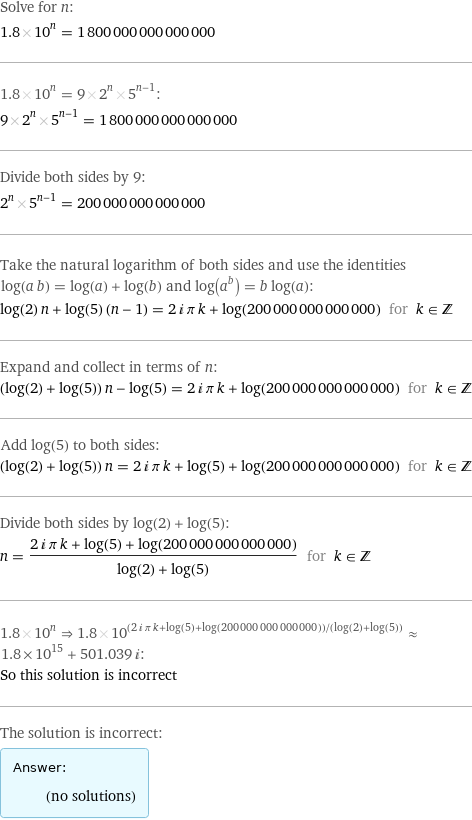Plot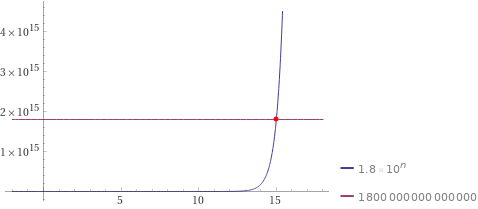Alternate forms assuming n is real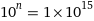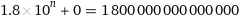Alternate formNumber line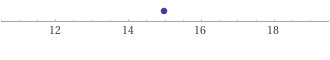Real solutions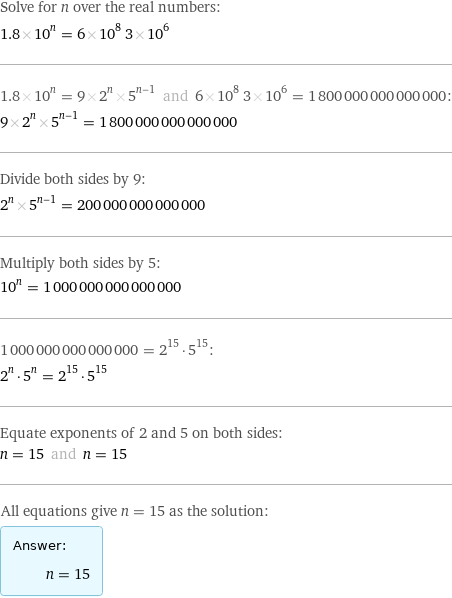Integer solutions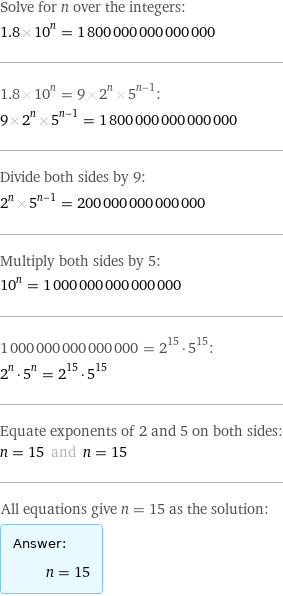Solution
View full explanation on CameraMath App.